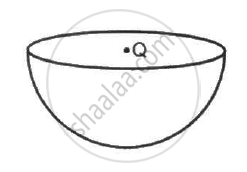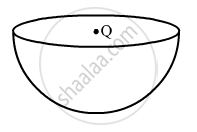Department of Pre-University Education, Karnataka course PUC Karnataka Science Class 12
Share

# A Charge Q is Placed at the Centre of an Imaginary Hemispherical Surface. Using Symmetry Arguments and Gauss'S Law - Physics

ConceptElectric Field Introduction of Electric Field

#### Question

A charge Q is placed at the centre of an imaginary hemispherical surface. Using symmetry arguments and Gauss's Law, find the flux of the electric field due to this charge through the surface of the hemisphere in the following figure.#### Solution

From Guass's law, flux through a closed surface, phi = "Q"_("e""n")/∈_0,

where
Qen = charge enclosed by the closed surface
Let us assume that a spherical closed surface in which the charge is enclosed is Q.
The flux through the sphere,

phi = "Q"/∈_0Hence for a hemisphere(open bowl), total flux through its curved surface,

phi′ = "Q"/∈_0 xx 1/2 = "Q"/(2∈_0)

Is there an error in this question or solution?

#### APPEARS IN

Solution A Charge Q is Placed at the Centre of an Imaginary Hemispherical Surface. Using Symmetry Arguments and Gauss'S Law Concept: Electric Field - Introduction of Electric Field.
S